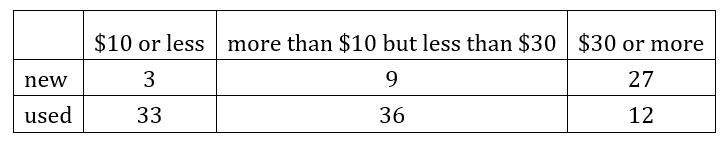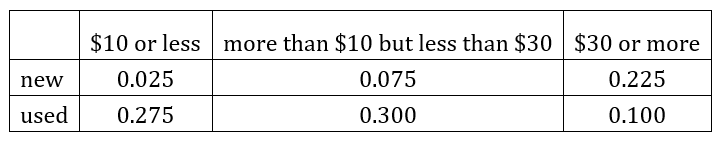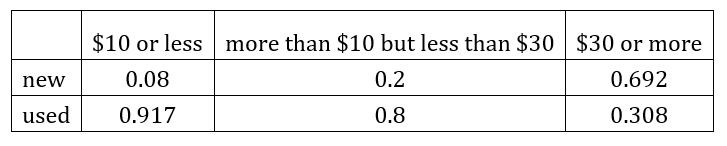# A.3.2 Lesson Summary

How do you create a relative frequency table?

Converting two-way tables to relative frequency tables can help reveal patterns in paired categorical variables. Relative frequency tables are created by dividing the value in each cell in a two-way table by the total number of responses in the entire table, or the total responses in a row or a column. Depending on what patterns are important, different types of relative frequency tables are used. To examine how individual combinations of the categorical variables relate to the whole group, divide each value in a two-way table by the total number of responses in the entire table to find the relative frequency. For example, this two-way table displays the condition of a certain textbook and its price for 120 of the books at a college bookstore.A two-way relative frequency table is created by dividing each number in the two-way table by 120, because there are 120 values (3 + 9 + 27 + 33 + 36 +12) in this data set. The resulting two-way relative frequency table can be represented using fractions or decimals.This two-way relative frequency table allows you to see what proportion of the total is represented by each number in the two-way table. The number 33 in the original two-way table represents the number of used books that also sell for \$10 or less, which is 27.5% of all the books in the data set. Using this two-way relative frequency table, you can see that there are very few (2.5%) new books that are also inexpensive and that 10% of the books in the bookstore are both expensive and in used condition. In other situations, it makes sense to examine row or column proportions in a relative frequency table. For example, to convert the original two-way table to a column relative frequency table using column proportions, divide each value by the sum of the column.This shows that about 91.7% (33/(3+33) is about 0.917) of the books that are sold for \$10 or less are in used condition. Notice that each column of this column relative frequency table reveals the proportions of the books in each price category that are in each condition and the relative frequencies in each column sum to 1. In particular, this shows that most of the inexpensive and moderately priced books are used, and most of the expensive books are new.